# The PLS Procedure

### Cross Validation

None of the regression methods implemented in the PLS procedure fit the observed data any better than ordinary least squares (OLS) regression; in fact, all of the methods approach OLS as more factors are extracted. The crucial point is that, when there are many predictors, OLS can overfit the observed data; biased regression methods with fewer extracted factors can provide better predictability of future observations. However, as the preceding observations imply, the quality of the observed data fit cannot be used to choose the number of factors to extract; the number of extracted factors must be chosen on the basis of how well the model fits observations not involved in the modeling procedure itself.

One method of choosing the number of extracted factors is to fit the model to only part of the available data (the training set) and to measure how well models with different numbers of extracted factors fit the other part of the data (the test set). This is called test set validation. However, it is rare that you have enough data to make both parts large enough for pure test set validation to be useful. Alternatively, you can make several different divisions of the observed data into training set and test set. This is called cross validation, and there are several different types. In one-at-a-time cross validation, the first observation is held out as a single-element test set, with all other observations as the training set; next, the second observation is held out, then the third, and so on. Another method is to hold out successive blocks of observations as test sets—for example, observations 1 through 7, then observations 8 through 14, and so on; this is known as blocked validation. A similar method is split-sample cross validation, in which successive groups of widely separated observations are held out as the test set—for example, observations {1, 11, 21, …}, then observations {2, 12, 22, …}, and so on. Finally, test sets can be selected from the observed data randomly; this is known as random sample cross validation.

Which validation you should use depends on your data. Test set validation is preferred when you have enough data to make a division into a sizable training set and test set that represent the predictive population well. You can specify that the number of extracted factors be selected by test set validation by using the CV= TESTSET(data set) option, where data set is the name of the data set containing the test set. If you do not have enough data for test set validation, you can use one of the cross validation techniques. The most common technique is one-at-a-time validation (which you can specify with the CV= ONE option or just the CV option), unless the observed data are serially correlated, in which case either blocked or split-sample validation might be more appropriate (CV= BLOCK or CV= SPLIT); you can specify the number of test sets in blocked or split-sample validation with a number in parentheses after the CV= option. Note that CV= ONE is the most computationally intensive of the cross validation methods, since it requires a recomputation of the PLS model for every input observation. Also, note that using random subset selection with CV= RANDOM might lead two different researchers to produce different PLS models on the same data (unless the same seed is used).

Whichever validation method you use, the number of factors chosen is usually the one that minimizes the predicted residual sum of squares (PRESS); this is the default choice if you specify any of the CV methods with PROC PLS. However, often models with fewer factors have PRESS statistics that are only marginally larger than the absolute minimum. To address this, van der Voet (1994) has proposed a statistical test for comparing the predicted residuals from different models; when you apply van der Voet’s test, the number of factors chosen is the fewest with residuals that are insignificantly larger than the residuals of the model with minimum PRESS.

To see how van der Voet’s test works, let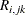be the jth predicted residual for response k for the model with i extracted factors; the PRESS statistic is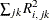. Also, let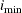be the number of factors for which PRESS is minimized. The critical value for van der Voet’s test is based on the differences between squared predicted residuals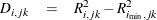One alternative for the critical value is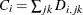, which is just the difference between the PRESS statistics for i andfactors; alternatively, van der Voet suggests Hotelling’s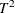statistic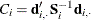, where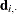is the sum of the vectors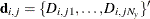and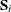is the sum of squares and crossproducts matrix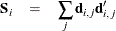Virtually, the significance level for van der Voet’s test is obtained by comparing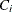with the distribution of values that result from randomly exchanging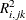and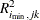. In practice, a Monte Carlo sample of such values is simulated and the significance level is approximated as the proportion of simulated critical values that are greater than. If you apply van der Voet’s test by specifying the CVTEST option, then, by default, the number of extracted factors chosen is the least number with an approximate significance level that is greater than 0.10.